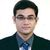# New to Qlik Sense

If you’re new to Qlik Sense, start with this Discussion Board and get up-to-speed quickly.

cancel
Showing results for
Did you mean:Contributor III

## trying to measure 2 conditions

hi,

count({<[Year]={\$(=max([Year])-1)}>}EMP_STATUS='A')

in the above statement i m trying to count emp_status value A for perivous year .

is it correct ?

1 Solution

Accepted SolutionsMVP

no,

Correct is as below.

count({<[Year]={"\$(=max([Year])-1)"},EMP_STATUS={"A"}>}EMP_STATUS)

Regards,

Kaushik Solanki

Regards,
Kaushik
12 RepliesMVP

no,

Correct is as below.

count({<[Year]={"\$(=max([Year])-1)"},EMP_STATUS={"A"}>}EMP_STATUS)

Regards,

Kaushik Solanki

Regards,
KaushikPartner

I don't thinkk that would be ok, I would do it as follow:

count({<EMP_STATUS={'A'},[Year]={\$(=max([Year])-1)}>}EMP_STATUS)Contributor III
Author

what if we want to calculate for the same yearContributor III
Author

what if we want to calculate for the same yearMVP

It will be

count({<EMP_STATUS={'A'},[Year]={\$(=max([Year]))}>}EMP_STATUS)

Regards,
KaushikPartner

it should looks like below:

count( {< EMP_STATUS = {'A'}, [Year] = {"\$(=Max([Year]))"}>} EMP_STATUS )Contributor III
Author

can i get tutorial/training guide for this ?Contributor III
Author

can i get tutorial/training guide for this ?Master II

HI,

PFA,